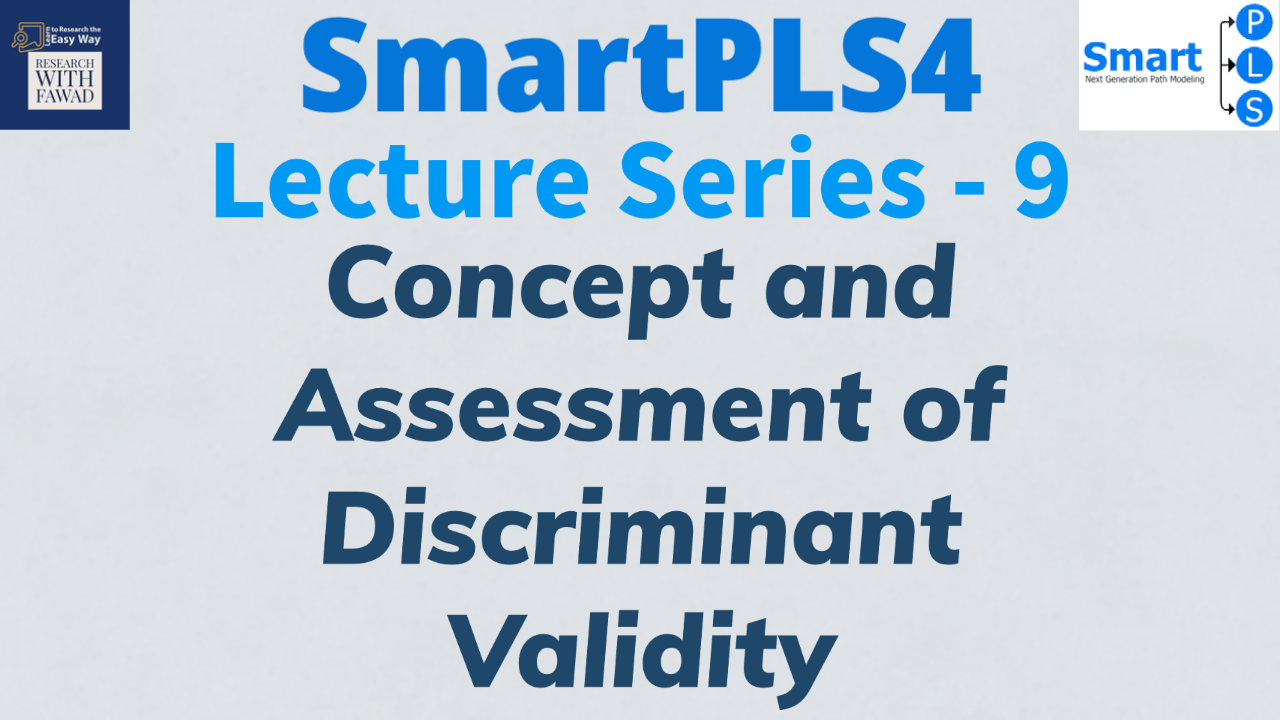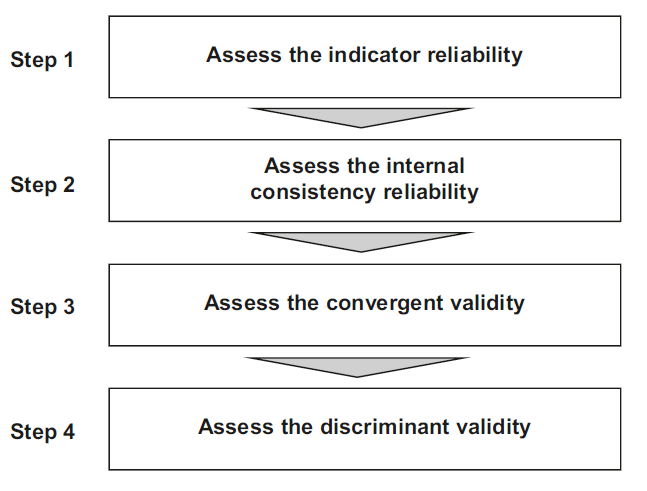# How to Assess Discriminant Validity?## Understanding Discriminant Validity.

This session discusses in detail the concept and assessment of Discriminant Validity using SmartPLS 4. The statistic is assessed as part of the measurement model. Discriminant validity is assessed using the following techniques

• Fornell & Larcker Criterion
• Heterotrait Monotrait Ratio

Most often, the constructs are reflective at lower level. Hence, all the lower order constructs in the study are assessed for reliability and validity. The following figure highlights the steps in measurement model assessment.In the last session we discussed how to assess Step 3 (Convergent Validity). In this tutorial, the focus is on convergent validity (Step 3).### What is Discriminant Validity?

The fourth step is to assess discriminant validity. This metric measures the extent to which a construct is empirically distinct from other constructs in the structural model.

### How to Establish Discriminant Validity?

To measure discriminant validity, there are three techniques

• Fornell & Larcker Criterion
• Heterotrait Monotrait Ratio

#### Fornell and Larcker Criterion

Fornell and Larcker (1981) proposed the traditional metric and suggested that each construct’s Square root of AVE (squared variance within) should be compared to the inter-construct correlation (as a measure of shared variance between constructs) of that same construct and all other reflectively measured constructs in the structural model – the shared variance between all model constructs should not be larger than their AVEs. Hence, a higher square root of AVE of a construct when compared to the correlation of that construct with all the other constructs in the study establishes discriminant validity.

#### Heterotrait-Monotrait (HTMT) Ratio

As a better alternative, we recommend the heterotrait–monotrait ratio (HTMT) of correlations (Henseler et al., 2015) to assess discriminant validity. The HTMT is defined as the mean value of the indicator correlations across constructs (i.e., the heterotrait–heteromethod correlations) relative to the (geometric) mean of the average correlations for the indicators measuring the same construct (i.e., the monotrait–heteromethod correlations).
Discriminant validity problems are present when HTMT values are high. Henseler et al. (2015) propose a threshold value of 0.90 for structural models with constructs that are conceptually very similar. When constructs are conceptually more distinct, a lower, more conservative, threshold value is suggested, such as 0.85 (Henseler et al., 2015).